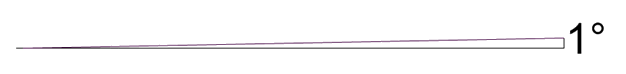# Measurement of angles in degrees, minutes and seconds

What defines an angle is the aperture of its sides. Therefore it is natural for us to wonder how should we measure this aperture. To measure an angle, what we do is comparing it with another that we use as a unit.

The most usual unit of measurement for angles is the sexagesimal degree, which consists in $$\frac{1}{360}$$ of a full angle. The measurement of an angle in sexagesimal degrees is denoted by the symbol $$^\circ$$.

An angle of $$56^\circ$$ is the one that has an aperture $$56$$ times larger than the opening of one degree (the sexagesimal unit).

To get an idea, one degree corresponds to the following aperture:So, in a full angle, which corresponds to a full circle, we have $$360^\circ$$ ($$360$$ degrees). That is to say:As we can see in the drawing, a full circle is divided into $$360$$ parts, each of them denoting one degree, which is designated as $$1^\circ$$. So, a full angle has $$360^\circ$$, a straight angle has $$180^\circ$$ and a right angle has $$90^\circ$$. The acute angles have less than $$90^\circ$$ and the obtuse ones more than $$90^\circ$$, but less than $$180^\circ$$.

According to its range, we can also name some specific angles.

• Congruent angles are those that have the same aperture,
• Complementary angles are those which measure $$90^\circ$$ if we add them up,
• Supplementary angles are those which measure $$180^\circ$$ if we add them up,
• Conjugated angles are those which measure $$360^\circ$$ if we add them up.

An angle of $$30^\circ$$ has a complementary angle of $$60^\circ$$, and a supplementary one of $$150^\circ$$ and a conjugate one of $$330^\circ$$.

But, what happens when we have an angle of less than $$1^\circ$$?

To be able to speak about angles that measure less than $$1^\circ$$, we use submultiples of a degree, so we avoid working with expressions like the following:

• This angle measures half a degree
• This angle measures $$0,76$$ degrees

Thus, the sexagesimal degree has submultiples: these are the minute and the second. The minute is designated as $$'$$ and the second as $$''$$.

The measurement of an angle in degrees, minutes and seconds would be, for example, $$84^\circ 17' \ 43 \ ''$$. It would be read as: an angle of $$84$$ degrees, $$17$$ minutes and $$43$$ seconds.

Let's see the exact value of minutes and seconds.

• One minute is the result of taking a degree and dividing it into $$60$$ equal parts. This is, mathematically expressed: $$1$$ minute $$= \dfrac{1^\circ}{60}$$ and therefore $$60$$ minuts $$= 1^\circ$$.
• A second is the result of taking a minute and dividing it in $$60$$ equal parts. This is, mathematically expressed: $$1$$ second $$= \dfrac{1'}{60}$$ and therefore $$60$$ seconds $$= 1$$ minut.

With this equivalence let's see the value of a degree in seconds:

$$\left. \begin{array}{rcl} 1^\circ & = & 60' \\\\ 1' & = & 60'' \end{array} \right\} \Longrightarrow 1^\circ= 60 \cdot 60 ''= 3600 ''$$$To change degrees into minutes and seconds we will always work by means of conversion factors. This means that we will use the following method: We want to write $$32^\circ$$ in minutes and $$21^\circ$$ in seconds. $$32^\circ = 32 \ \mbox{degrees} \cdot \dfrac {60 \ \mbox{minutes}}{1 \ \mbox{degree}} = 32 \cdot 60 \ \mbox{minutes} = 1920 \ \mbox{minutes}$$$

In other words, we know that $$60$$ minutes $$= 1^\circ$$, therefore $$\dfrac{60 \ \mbox{minutes}}{1^\circ}=1$$ and, through this conversion factor, we change from degrees to minutes.

We do the same in the case of seconds. Knowing that $$60 \ \mbox{seconds}=1 \ \mbox{minute}$$, if we move the term on the right-hand side onto the left-hand side to divide it, we get $$\dfrac{60 \ \mbox{seconds}}{1 \ \mbox{minute}}=1$$, which is the conversion factor to change from minutes to seconds. By this method,

$$21^\circ = 21^\circ \cdot \frac{60 \ \mbox{minutes}}{1 ^\circ} \cdot \frac{60 \ \mbox{seconds}}{1 \ \mbox{minute}} = 21 \cdot 60 \cdot 60 \ \mbox{seconds}= 75600 \ \mbox{seconds}$$$Finally, we will see an example that allows us to express in degrees quantities given in seconds or minutes. If we have $$460$$ seconds, then we have: $$39600 \ \mbox{seconds}= 39600 \ \mbox{seconds} \dfrac{1 \ \mbox{minute}}{60 \ \mbox{seconds}} = \dfrac {39600}{60} \ \mbox{minutes} = 660 \ \mbox{minutes}$$$

If we want to express it in degrees: $$39600 \ \mbox{seconds}=\dfrac {39600}{60} \ \mbox{minutes} \cdot \dfrac{1 \ \mbox{degree}}{60 \ \mbox{minutes}} = \dfrac {39600}{60·60} \ \mbox{degrees} = 11 \ \mbox{degrees}$$\$

## Measuring drawn angles

Angles can be measured by means of tools such as the goniometer, the quadrant, the sextant, the cross-staff or the protractor.

The most common is the protractor, which is a drawing tool that allows us not only to measure but also to construct angles.

It consists of a graduated half-disk with which we can measure angles of up to $$180^\circ$$.